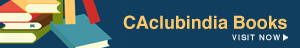# Understanding Foreign Exchange - Part - 2

Aravind.. , 19 July 2011

-series of articles on foreign exchange-

My last article was about quoting of foreign exchanges in different ways. This article is the continuation of the last one. Quoting of one currency against another is not done at same rate for buying and selling. A dollar cannot be both bought and sold for same amount of rupees at any point of time.

1\$ = 47.5 – 47.75

The above is a direct quote in terms of Indian rupees. Now we have to understand this quote. Always there are two rates in the foreign exchange market. They are bid rate or buying rate and ask rate or selling rate. In any quote the smaller amount at the left side is called bid rate and the larger one at the right side is called the ask rate. In the given quote,

Bid rate = 47.5, Ask rate = 47.75.

Bid rate is the rate at which a bank buys a currency, whereas ask rate is the rate at which it sells the currency. RBI buys an USD by giving INR.47.5 and sells an USD for INR 47.75.

Why there are two rates? The answer is, RBI which maintains the forex market and performs all other functions for stabilizing the currency movements, needs a return. The difference between the buying rate and selling rate is the benefit for RBI.

Let us now understand the mechanism of bid and ask rates. Bid rate is the rate at which bank buys a currency and ask rate the vice versa. This is exactly the opposite in the market. Bid rate is the rate at which a person can sell a currency to the bank and ask rate is the rate at which a person can buy currency from the bank.

The action is simple,

Bank can buy only when someone sells to the bank  -  BID RATE.

Bank can sell only when someone buys from the bank  -  ASK RATE.

So, we can buy one dollar by giving INR 47.75 and sell a dollar and get INR 47.5.

The difference between Bid rate and Ask rate is called as Spread. Therefore it is the benefit that accrues to the bank.

In this type of two way quoting, direct quote can be converted into indirect quote by the following formula,

Price/ Product = INR/\$ = 47.5 – 47.75 – Direct Quote    Spread = 47.75 – 47.5 = 0.25

For converting the formula is,

Bid rate = 1/ ask rate.

Ask rate = 1/ Bid rate.

So, \$/INR = 1/47.75 – 1/47.5

\$/INR = 0.0209 – 0.0210  - Indirect quote.

So, that about Bid and ask rate, spread and how to convert direct quote into indirect quote by using bid and ask rate.

D.Aravind (SRO 0266513)

Aravind..
(CA)
Category Students   Report

44 Shares   12936 Views

### Related Articles### Popular ArticlesCCI Articles